# Circles and its Related Terms | Class 9 Maths

• Difficulty Level : Easy
• Last Updated : 21 Jul, 2021

Many objects that we encounter in our lifestyle are ‘round’ in shape like a coin, bangles, bottle caps, the Earth, wheels etc. In layman terms, the shape is usually mentioned as a circle. A closed plane figure, which is formed by the set of all those points which are equidistant from a fixed point in the same plane, is known as a circle.

The collection of all the points during a plane, which are at a hard and fast distance from a hard and fast point within the plane, is named a circle. In other words, a circle can be described as the locus of a point moving in a plane, in such a way that its distance from a fixed point is always constant. A closed plane figure, which is formed by the set of all those points which are equidistant from a fixed point in the same plane, is known as a circle.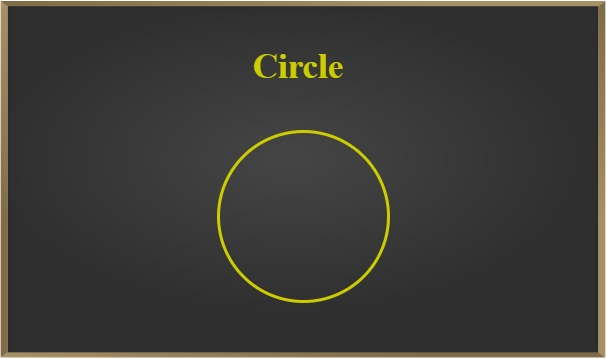### Circumference of Circle

The circumference of a circle is often defined as the distance around a circle. This can be understood with the help of an example. Suppose a wire of length 5 m is bent in order that it forms a circle. Here, the circumference is equal to the length of the wire, i.e. 5 m. The length of the entire circle is named its circumference.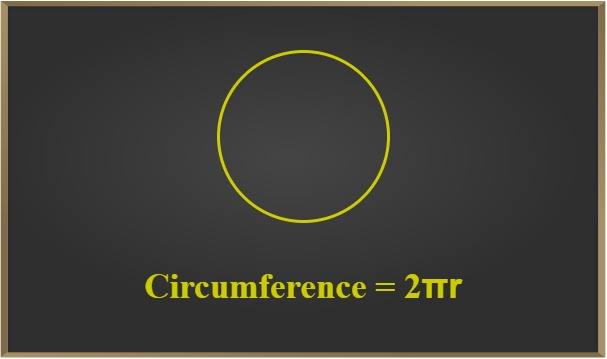Example: The radius of a circular rug is 4 ft. What is the circumference?

Solution:

radius = 4 ft

Circumference Formula = 2πr

=> 2 * 3.14 * 4 = 25.12ft

### Centre

A circle may be a curve all of whose points dwell an equivalent plane and are at an equivalent distance from the centre. The fixed point is called the centre of the circle. The above figure shows the green color dot called the centre.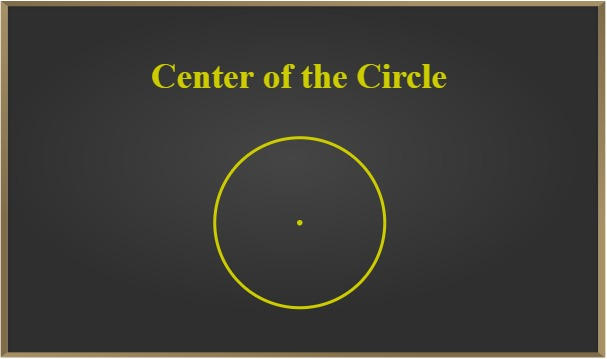The fixed point is named the centre of the circle and therefore the fixed distance is named the radius of the circle. The constant distance between any point on the circle and its centre is called the radius. Note that the line segment joining the centre and any point on the circle is additionally called a radius of the circle.

Radius = Diameter/2 = d/2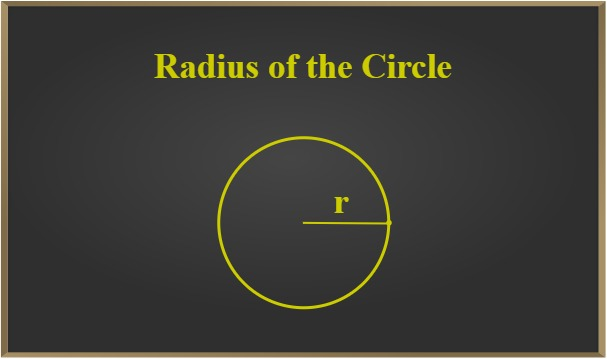Example: The distance around a carousel is 21.98 yd. What is the radius?

Solution:

Circumference = 21.98 yd

Diameter = 21.98 yd ÷ 3.14 = 7 yd

By Formula, Radius = Diameter/2

Therefore,

radius = 3.5 yd

### Diameter

A chord passing through the centre of the circle is called the diameter of the circle. Two radii at 180 degrees angle are the diameter of the circle. Diameter is the largest chord of the circle and every diameter has an equivalent length, which is adequate to twice the radius.

Diameter = 2 × radius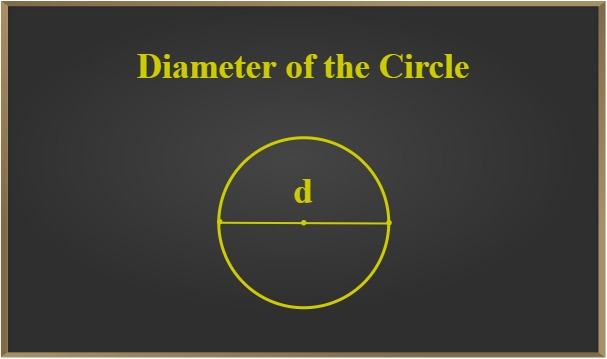Example: If the diameter of a circle is 142.8 mm, then what is the radius?

Solution:

diameter = 142.8 mm

By Formula, diameter = 2 * r

radius = (142.8 ÷ 2)= 71.4 mm

### Interior and Exterior of a Circle

A circle divides the plane on which it lies into three parts. They are:

• Inside the circle, which is additionally called the inside of the circle. Point lying in the plane of the circle such that its distance from its centre is less than the radius of the circle is known as the interior point.
• On the circle and Points lying in the plane of the circle such that its distance from its centre is equal to the radius of a circle. In simple words, a set of points lying on the circle are points on the circumference of a circle.
• Outside the circle, which is additionally called the outside of the circle. Points lying in the plane of the circle such that its distance from its centre is greater than the radius of the circle are exterior points.

Area of Track = π × (Outer Radius – Inner Radius)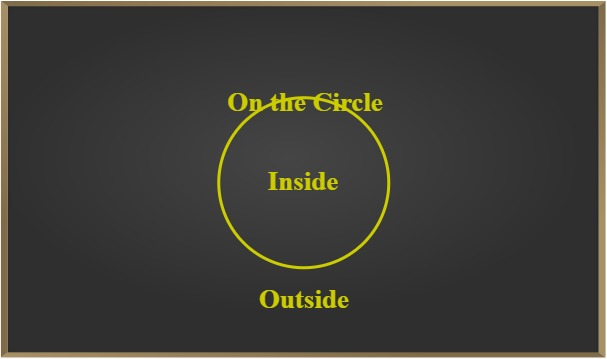Example: The inner circumference of a circular track is 440 m, and the track is 14 m wide. Calculate the
cost of levelling the track at 25 paise/m2.

Solution:

Let the radius of the inner circle be r m.

Now,

Inner circumference = 440 m

⇒ 2πr = 440

⇒ 2 × 22/7 × r = 440

⇒ r = 440 × 744

⇒ Inner radius, r = 70

We know that the track is 14 m wide.

∴ Outer radius (R) = (70 + 14) = 84 m

Area of the track = π(R− r)= π (842 – 702)

=> 22/7 × (7056 – 4900)

=> 6776 m2

Cost of levelling at 25 paise per square meter

=> 6676 × 25 = 169400 paise

or,

169400/100 = Rs.1694

### Chord

A line segment joining two different points on the circumference of a circle is called a chord of the circle. A circle can have any number of chords. Diameter is the largest chord of a circle. A chord of a circle may be a line joining two points of the circumference. A chord passes through the centre is named diameter. Note that Diameter is that the longest chord and every diameter has an equivalent length, which is adequate to twice the radius.

Length of Chord = 2 × √(radius2 − distance2)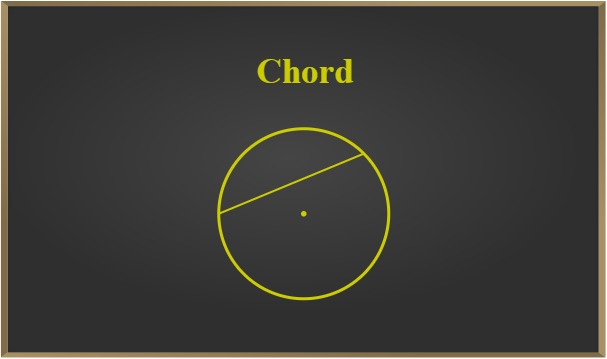Example: Find the length of the chord of a circle where the radius is 7 cm and perpendicular distance from
the chord to the centre is 4 cm?

Solution:

Given radius, r = 8 cm

and distance, d = 3 cm

Chord length = 2√(r2 – d2

⇒ Chord length = 2√(82 – 32

⇒ Chord length = 2√(64 – 9)

⇒ Chord length = 2√55

⇒ Chord length = 2 × 7.416

or, chord length = 14.83 cm

### Arcs

A piece of a circle between two points is named an arc. A part of the circumference of the circle is known as an arc. An arc is a continuous piece of the circle. The arc upper AB arc is known as the minor arc and the lower AB arc is the major arc. Now go through the circular region which is cut off from the rest of the circle by a secant or a chord. In a circle, equal chords have equal arcs.

Length of Arc = 2 * π * r * angle / 360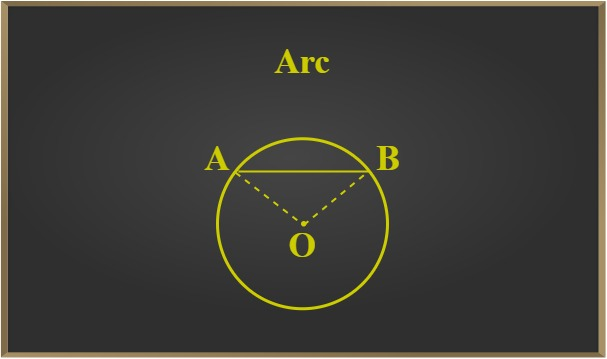Example: If the radius of a circle is 5 cm and the measure of the arc is 110˚, what is the length of the arc?

Solution:

Arc length = 2 * π * r * angle / 360

=> 2 * 3.14 * 5 * 110/360°

=> 9.6 cm

### Segment

The region between a chord and either of its arcs is named segment of the circle. Part of a circle bounded by a chord and an arc is known as a segment of the circle. The figure given below depicts the major and minor segments of the circle. Here, the segment AQBA is with major arc AQB is called Major Segment while the segment APBA with minor arc APB is called minor segment.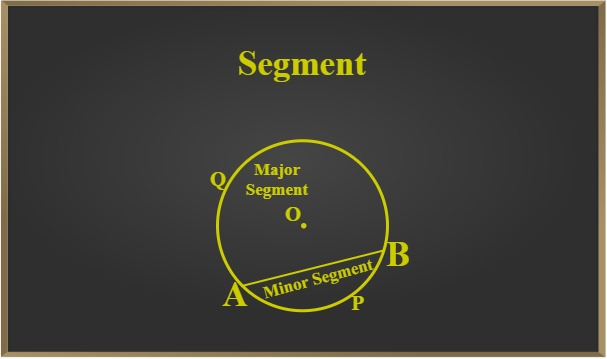Example: Find the lengths of the arcs cut off from a circle of radius 12 cm by a chord 12 cm long. Also,
find the area of the minor segment.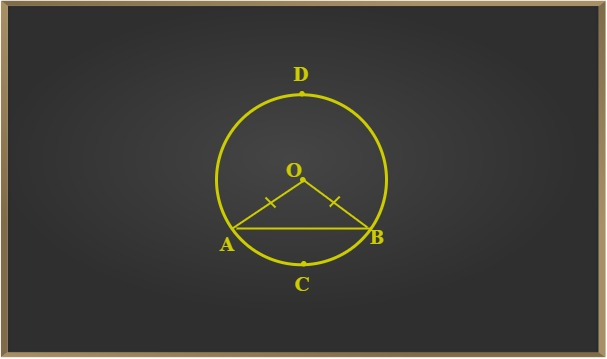Solution:

Let AB be the chord. Joining A and B to O, we get an equilateral triangle OAB.

Thus, we have: ∠O = ∠A = ∠B = 60°

Length of the arc ACB: 2π × 12 × 60/360 = 4π = 12.56 cm

Length of the arc ADB:

Circumference of the circle – Length of the arc ACB

=> 2π × 12 – 4π = 20π cm = 62.80 cm

Now, Area of the minor segment:

Area of the sector – Area of the triangle

=> [π × (12)2 × 60/360 – 3√4 × (12)2] = 13.08 cm2

### Sector

The region between an arc and therefore the two radii, joining the centre to the top points of the arc is named a sector. A sector of a circle is the part bounded by two radii and an arc of a circle. In the below-given fig. AOB is a sector of a circle with O as the centre. The minor arc corresponds to the minor sector and therefore the major arc corresponds to the main sector. When two arcs are equal, that is, each may be a semicircle, then both segments and both sectors become equivalent and each is understood as a semicircular region.

Area of sector = θ/360 × πr2Example: The area of a sector with a radius of 6 cm is 35.4 cm2. Calculate the angle of the sector.

Solution:

Area of sector = Angle * π * r * r/360°

Angle * π * 6 * 6/360° = 35.4

Angle = 35.4/36π × 360°

= 112.67°

### Some Problems with Solution

Question 1. Every diameter of a circle is additionally a chord. Is that the converse of this statement also true?

Answer: Every diameter could also be a chord because its endpoints lie on the circumference of the circle. It’s the longest chord that passes through the centre of the circle.

Question 2. State difference between sector and segment of a circle.

Answer: A sector is that the region between an arc and thus the 2 radii, joining the centre to the highest points of the arc whereas a segment is that the region between a chord of a circle and its associated arc.

Question 3. For a quadrilateral to become cyclic quadrilateral, sum of a pair of its opposite angles must be adequate to ________.

Answer: For a quadrilateral to become cyclic quadrilateral, sum of a pair of its opposite angles must be adequate to 180°.

Question 4. What percentage circles are often drawn passing through three non-collinear points?

Answer: One and only one circle can be drawn through three given non-collinear points.

My Personal Notes arrow_drop_up